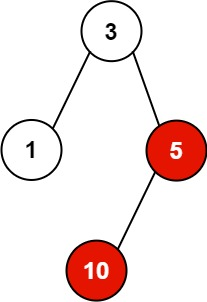### LeetCode• ㊗️
• 大家
• offer
• 多多！

## Problem

You have n processes forming a rooted tree structure. You are given two integer arrays pid and ppid, where pid[i] is the ID of the ith process and ppid[i] is the ID of the ith process’s parent process.

Each process has only one parent process but may have multiple children processes. Only one process has ppid[i] = 0, which means this process has no parent process (the root of the tree).

When a process is killed, all of its children processes will also be killed.

Given an integer kill representing the ID of a process you want to kill, return a list of the IDs of the processes that will be killed. You may return the answer in any order.

Example 1:Input: pid = [1,3,10,5], ppid = [3,0,5,3], kill = 5
Output: [5,10]
Explanation: The processes colored in red are the processes that should be killed.


Example 2:

Input: pid = , ppid = , kill = 1
Output: 


Constraints:

• n == pid.length
• n == ppid.length
• $1 <= n <= 5 * 10^4$
• $1 <= pid[i] <= 5 * 10^4$
• $0 <= ppid[i] <= 5 * 10^4$
• Only one process has no parent.
• All the values of pid are unique.
• kill is guaranteed to be in pid.

## Code

class Solution {
public List<Integer> killProcess(List<Integer> pid, List<Integer> ppid, int kill) {
// parent: a list of child
HashMap<Integer, List<Integer>> map = new HashMap<>();

for(int i = 0; i < pid.size(); i++){
int parent = ppid.get(i);
int child = pid.get(i);
if(!map.containsKey(parent)){
map.put(parent, new ArrayList<>());
}

}

List<Integer> res = new ArrayList<>();

dfs(res, map, kill);
return res;
}

private void dfs(List<Integer> res, HashMap<Integer, List<Integer>> map, int kill){